# ORELA Math: Calculating Derivatives & Derivative Rules Chapter Exam

Exam Instructions:

Choose your answers to the questions and click 'Next' to see the next set of questions. You can skip questions if you would like and come back to them later with the yellow "Go To First Skipped Question" button. When you have completed the practice exam, a green submit button will appear. Click it to see your results. Good luck!

### Page 1

#### Question 1 1. Find y'.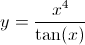#### Question 2 2. The derivative below may have been calculated from which function f(x)?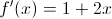#### Question 3 3. Evaluate.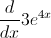#### Question 4 4. What is the derivative of f(x)?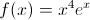#### Question 5 5. Find the derivative of f(x).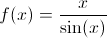### Page 2

#### Question 6 6. Evaluate the following: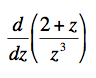#### Question 7 7. What is the derivative of f(x)?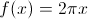#### Question 8 8. Find the derivative of f(x).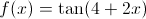#### Question 9 9. What is the derivative of f(z)?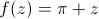#### Question 10 10. What is the derivative of f(x)?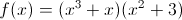### Page 3

#### Question 11 11. Find the derivative df/dt, given the function f(t).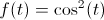#### Question 12 12. What is the derivative of y?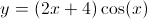#### Question 13 13. Which of the following statements is true about the derivative of f(x)?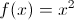#### Question 14 14. Calculate the derivative of g(t).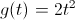#### Question 15 15. What is the derivative of f(t)?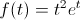### Page 4

#### Question 16 16. Find the derivative of f(x).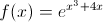#### Question 17 17. Differentiate the following function: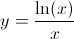#### Question 18 18. What is the derivative of f(x)?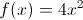#### Question 19 19. What is the derivative of y?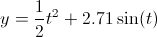#### Question 20 20. Find f'(x) for the following function.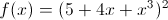### Page 5

#### Question 21 21. What is the derivative of g(t)? Assume C is a constant.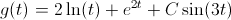#### Question 22 22. Find f'(t) given the function f(t):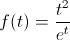#### Question 23 23. Which of the following statements is true, given g(t) below?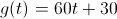#### Question 24 24. Evaluate the following.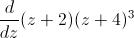#### Question 25 25. Calculate the derivative of f(x), and evaluate it at x=2.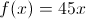#### ORELA Math: Calculating Derivatives & Derivative Rules Chapter Exam Instructions

Choose your answers to the questions and click 'Next' to see the next set of questions. You can skip questions if you would like and come back to them later with the yellow "Go To First Skipped Question" button. When you have completed the practice exam, a green submit button will appear. Click it to see your results. Good luck!

Support